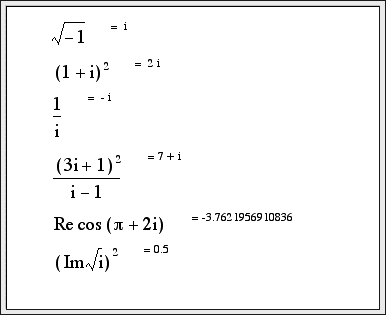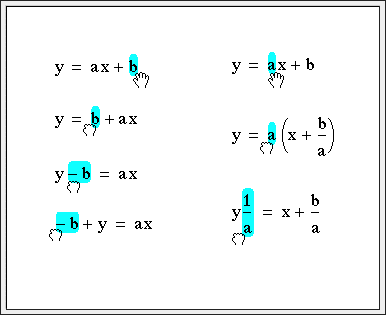## Equation Calculator

##### for Macintosh

Equation Calculator is a scientific calculator which does symbolic and algebraic manipulation, algebra and calculus as well as numeric computation. You can define variables and functions, evaluate symbolic derivatives, numeric integrals and matrix operations.

#### Features

• Scientific Calculator
• Simplify expressions
• Evaluate derivatives
• Numeric integration
• Complex numbers
• Drag terms with the mouse to transform expressions
• Isolate terms symbolically
• Define variables
• Trigonometric and logarithmic functions
• Bessel functions
• Floor, ceiling, min, max, mod
• Piecewise defined functions
• Symbolic summations
• Symbolic substitutionsComplex numbersDrag algebra

Buy Equation Calculator on the Mac App Store.